Related Articles

# PointNet – Deep Learning

• Last Updated : 08 Jun, 2021

PointNet was proposed by a researcher at Stanford University in 2016. The motivation behind this paper is to classify and segment 3D representation of images. They use a data structure called Point cloud, which is a set of the point that represents a 3D shape or an object. Due to its irregularities, it is only suitable for a particular use case.

Many authors converted the point cloud into some other representation called voxel (volumetric pixel) before it is fed into the Deep neural networks. However, such transformation leads data too voluminous, and introducing quantization to the 3D structure can also lead to variance from natural artifacts.

In this paper, the author proposes a novel method for directly consuming Point clouds and output the relevant classification of image or segmentation.

### Architecture

The author proposes an architecture that takes Point Sets from Point cloud as input. The point cloud is represented by a set of 3D-points Pi where each point is represented as(xi, yi, zi).

For the object classification task, the input point cloud is directly sampled from the shape or pre-segmented from the scene point cloud. For semantic segmentation, the input can be a single object from the part region segmentation or a small part of 3D scene from the Object region segmentation.

Some properties of Point Sets are:

• Permutation Invariance: Since the points in the point cloud is unstructured, a scan of N points has N! different permutations. The data processing must be invariant to different permutated representations of Point Cloud.
• Transformation invariance: The classification and segmentation output can not be impacted by different transformations like rotation and translation.
• Interaction b/w different Points: The connections between neighboring points often carries useful information. Therefore, each point should not be treated in isolation. These interactions can play a more useful role in segmentation than classification.

PointNet architecture

The PointNet architecture is quite intuitive. The classification network uses a shared multi-layer perceptron to map each of the n points from 3 dimensions to 64-dimension. It’s important that a single multi-layer perceptron is shared for each of the n points. Similarly, in the next layer, each n point is mapped from 64 dimensions to 1024 dimensions. Now, we apply max-pooling to create a global feature vector in ℝ¹⁰²⁴. Finally, a three-layer fully-connected network (FCNs) is used to map the global feature vector to k output classification scores.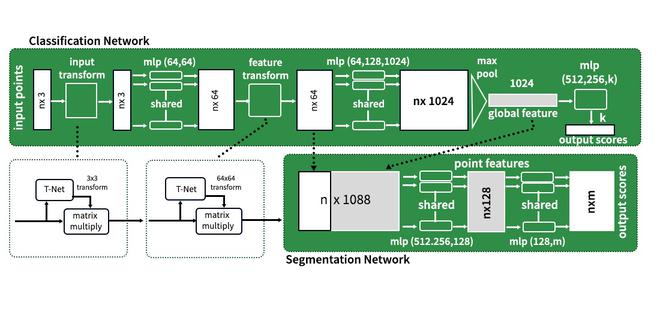Pointnet architecture

For the segmentation network, each of the n inputs needs to assign, one of the m segmentation classes, because segmentation relies on local and global features, the points in the 64-dimensional space are concatenated with the global feature space, resulting in possible feature space of n * ℝ¹⁰88.

The PointNet architecture has these key modules: the max-pooling layer, a local and global combination structure, and two joint alignment networks that align both local and global networks. Similar to per point

#### Symmetry function

To make a model invariant from the permutation, three strategies exist:

• Sort input into canonical order.
• Treat the input as a sequence to train the RNN
• Use a simple symmetric function to aggregate the information from each point.

Below is an example of symmetric functionwhereh here can be multi-layer perceptron, g is a composition of single variable function and a max-pooling function and f can be the output layer.

#### Local and Global Information Aggregation

The output from the above section forms a vector [f1, f2, ….fn], i.e the global signature of the input set. Now, this will work fine as we can easily train the SVM to make a classifier output. But, for the point segmentation, we require a combination of both local and global features.

To get the desired result, after computing the global feature vector the authors feed it back to the point feature by concatenating global features with per point features (see in the above image of architecture). This method is able to predict per point quantities that relies both on global semantics and local features

#### Joint Alignment Network

The semantic labeling of the point cloud has to be geometric transformation invariant (i.e. invariant of any rotation, translation etc.). The author uses a mini-network to predict the affine transformation matrix and applies this transformation to the coordinates of the input point.

In the final step of T-net, The input dependent features at the final fully connected layer of T-net are combined with globally trainable weights and biases resulting in a 3×3 transformation matrix.

The concept of pose normalization extended to 64-d embedding space. The T-net is similar to the above figure except for nearly, except for an increase in the dimensionality of trainable weights and biases which becomes 256*4096*4096 respectively returning in a 64*64 transformation matrix. The increased number of trainable leads to overfitting, that’s why the authors introduced a regularization term that encourages the resulting 64*64 transformation.## Python3

 # codeimport osimport datetimeimport globimport trimeshimport numpy as npimport tensorflow as tffrom tensorflow import kerasfrom tensorflow.keras import layersimport matplotlib.pyplot as plt tf.random.set_seed(1) # Load modelDATA_DIR = tf.keras.utils.get_file(    "modelnet.zip",    "http://3dvision.princeton.edu/projects/2014/3DShapeNets/ModelNet10.zip",    extract=True,)DATA_DIR = os.path.join(os.path.dirname(DATA_DIR), "ModelNet10") mesh = trimesh.load(os.path.join(DATA_DIR, "chair/train/chair_0001.off")) # sample point frompoints = mesh.sample(2048)fig = plt.figure(figsize=(5, 5))ax = fig.add_subplot(111, projection="3d")ax.scatter(points[:, 0], points[:, 1], points[:, 2],color = 'red')ax.set_axis_off()plt.show() # function to parse datasetdef parse_dataset(num_points=2048):     train_points = []    train_labels = []    test_points = []    test_labels = []    class_map = {}    folders = glob.glob(os.path.join(DATA_DIR, "[!README]*"))     for i, folder in enumerate(folders):        print("processing class: {}".format(os.path.basename(folder)))        # store folder name with ID so we can retrieve later        class_map[i] = folder.split("/")[-1]        # gather all files        train_files = glob.glob(os.path.join(folder, "train/*"))        test_files = glob.glob(os.path.join(folder, "test/*"))         for f in train_files:            train_points.append(trimesh.load(f).sample(num_points))            train_labels.append(i)         for f in test_files:            test_points.append(trimesh.load(f).sample(num_points))            test_labels.append(i)     return (        np.array(train_points),        np.array(test_points),        np.array(train_labels),        np.array(test_labels),        class_map,    ) class OrthogonalRegularizer(keras.regularizers.Regularizer):    def __init__(self, num_features, l2reg=0.001):        self.num_features = num_features        self.l2reg = l2reg        self.eye = tf.eye(num_features)     def __call__(self, x):        x = tf.reshape(x, (-1, self.num_features, self.num_features))        xxt = tf.tensordot(x, x, axes=(2, 2))        xxt = tf.reshape(xxt, (-1, self.num_features, self.num_features))        return tf.reduce_sum(self.l2reg * tf.square(xxt - self.eye))# Create the T-net modeldef t_net(inputs, num_features):     # Initialise bias as the indentity matrix    bias = keras.initializers.Constant(np.eye(num_features).flatten())    reg = OrthogonalRegularizer(num_features)     x = conv_bn(inputs, 32)    x = conv_bn(x, 64)    x = conv_bn(x, 512)    x = layers.GlobalMaxPooling1D()(x)    x = dense_bn(x, 256)    x = dense_bn(x, 128)    x = layers.Dense(        num_features * num_features,        kernel_initializer="zeros",        bias_initializer=bias,        activity_regularizer=reg,    )(x)    feat_T = layers.Reshape((num_features, num_features))(x)    # Apply affine transformation to input features    return layers.Dot(axes=(2, 1))([inputs, feat_T]) # the main modelinputs = keras.Input(shape=(NUM_POINTS, 3)) x = t_net(inputs, 3)x = conv_bn(x, 32)x = conv_bn(x, 32)x = t_net(x, 32)x = conv_bn(x, 32)x = conv_bn(x, 64)x = conv_bn(x, 512)x =layers.GlobalMaxPooling1D()(x)x = dense_bn(x, 256)x = layers.Dropout(0.3)(x)x = dense_bn(x, 128)x = layers.Dropout(0.3)(x) outputs = layers.Dense(NUM_CLASSES, activation="softmax")(x) model = keras.Model(inputs=inputs, outputs=outputs, name="pointnet")model.summary() %load_ext tensorboard # compile and train the modelmodel.compile(    loss="sparse_categorical_crossentropy",    optimizer=keras.optimizers.Adam(learning_rate=0.001),    metrics=["sparse_categorical_accuracy"],)log_dir = "logs/fit/" + datetime.datetime.now().strftime("%Y%m%d-%H%M%S")tensorboard_callback = tf.keras.callbacks.TensorBoard(log_dir=log_dir, histogram_freq=1)print(log_dir)model.fit(train_dataset, epochs=30, validation_data=test_dataset,          callbacks=[tensorboard_callback])
logs/fit/20210309-060624
WARNING:tensorflow:Model failed to serialize as JSON. Ignoring... <__main__.OrthogonalRegularizer object at 0x7fc3ecd25790> does not implement get_config()
Epoch 1/30
125/125 [==============================] - 36s 251ms/step - loss: 3.9923 - sparse_categorical_accuracy: 0.2046 - val_loss: 43220470648012800.0000 - val_sparse_categorical_accuracy: 0.2687
Epoch 2/30
125/125 [==============================] - 30s 239ms/step - loss: 3.1246 - sparse_categorical_accuracy: 0.3611 - val_loss: 12.8184 - val_sparse_categorical_accuracy: 0.2137
Epoch 3/30
125/125 [==============================] - 30s 239ms/step - loss: 2.8952 - sparse_categorical_accuracy: 0.4318 - val_loss: 3.3341 - val_sparse_categorical_accuracy: 0.1707
Epoch 4/30
125/125 [==============================] - 30s 239ms/step - loss: 2.6418 - sparse_categorical_accuracy: 0.4795 - val_loss: 268835504128.0000 - val_sparse_categorical_accuracy: 0.4747
Epoch 5/30
125/125 [==============================] - 30s 239ms/step - loss: 2.5744 - sparse_categorical_accuracy: 0.5262 - val_loss: 1399391744.0000 - val_sparse_categorical_accuracy: 0.5165
Epoch 6/30
125/125 [==============================] - 30s 239ms/step - loss: 2.3542 - sparse_categorical_accuracy: 0.6136 - val_loss: 911933.9375 - val_sparse_categorical_accuracy: 0.5936
Epoch 7/30
125/125 [==============================] - 30s 239ms/step - loss: 2.2442 - sparse_categorical_accuracy: 0.6602 - val_loss: 257217894776045568.0000 - val_sparse_categorical_accuracy: 0.6410
Epoch 8/30
125/125 [==============================] - 30s 238ms/step - loss: 2.1114 - sparse_categorical_accuracy: 0.6685 - val_loss: 50140152856576.0000 - val_sparse_categorical_accuracy: 0.6960
Epoch 9/30
125/125 [==============================] - 30s 239ms/step - loss: 2.0264 - sparse_categorical_accuracy: 0.6971 - val_loss: 117848482353512448.0000 - val_sparse_categorical_accuracy: 0.7159
Epoch 10/30
125/125 [==============================] - 30s 239ms/step - loss: 2.0393 - sparse_categorical_accuracy: 0.6928 - val_loss: 2660748754944.0000 - val_sparse_categorical_accuracy: 0.6322
Epoch 11/30
125/125 [==============================] - 30s 239ms/step - loss: 1.9129 - sparse_categorical_accuracy: 0.7376 - val_loss: 20.5381 - val_sparse_categorical_accuracy: 0.7048
Epoch 12/30
125/125 [==============================] - 30s 238ms/step - loss: 1.8221 - sparse_categorical_accuracy: 0.7659 - val_loss: 534893165459537920.0000 - val_sparse_categorical_accuracy: 0.7148
Epoch 13/30
125/125 [==============================] - 30s 239ms/step - loss: 1.7931 - sparse_categorical_accuracy: 0.7741 - val_loss: 14077352313094144.0000 - val_sparse_categorical_accuracy: 0.7192
Epoch 14/30
125/125 [==============================] - 30s 239ms/step - loss: 1.7970 - sparse_categorical_accuracy: 0.7683 - val_loss: 9279.2363 - val_sparse_categorical_accuracy: 0.7808
Epoch 15/30
125/125 [==============================] - 30s 239ms/step - loss: 1.7285 - sparse_categorical_accuracy: 0.7924 - val_loss: 8201817088.0000 - val_sparse_categorical_accuracy: 0.8304
Epoch 16/30
125/125 [==============================] - 30s 238ms/step - loss: 1.7426 - sparse_categorical_accuracy: 0.7912 - val_loss: 1834421736964096.0000 - val_sparse_categorical_accuracy: 0.7555
Epoch 17/30
125/125 [==============================] - 30s 238ms/step - loss: 1.6427 - sparse_categorical_accuracy: 0.8237 - val_loss: 309827239936.0000 - val_sparse_categorical_accuracy: 0.7610
Epoch 18/30
125/125 [==============================] - 30s 238ms/step - loss: 1.6883 - sparse_categorical_accuracy: 0.8182 - val_loss: 12362231232444401451008.0000 - val_sparse_categorical_accuracy: 0.6740
Epoch 19/30
125/125 [==============================] - 30s 238ms/step - loss: 1.6198 - sparse_categorical_accuracy: 0.8378 - val_loss: 168301294885625921536.0000 - val_sparse_categorical_accuracy: 0.7048
Epoch 20/30
125/125 [==============================] - 30s 238ms/step - loss: 1.6321 - sparse_categorical_accuracy: 0.8265 - val_loss: 34155740306341888.0000 - val_sparse_categorical_accuracy: 0.7963
Epoch 21/30
125/125 [==============================] - 30s 238ms/step - loss: 1.6206 - sparse_categorical_accuracy: 0.8237 - val_loss: 73268587348667400192.0000 - val_sparse_categorical_accuracy: 0.7874
Epoch 22/30
125/125 [==============================] - 30s 238ms/step - loss: 1.5612 - sparse_categorical_accuracy: 0.8497 - val_loss: 1441606803694551040.0000 - val_sparse_categorical_accuracy: 0.8007
Epoch 23/30
125/125 [==============================] - 30s 238ms/step - loss: 1.6024 - sparse_categorical_accuracy: 0.8288 - val_loss: 672064995328.0000 - val_sparse_categorical_accuracy: 0.8249
Epoch 24/30
125/125 [==============================] - 30s 238ms/step - loss: 1.5145 - sparse_categorical_accuracy: 0.8572 - val_loss: 416892130609315446784.0000 - val_sparse_categorical_accuracy: 0.8040
Epoch 25/30
125/125 [==============================] - 30s 239ms/step - loss: 1.5235 - sparse_categorical_accuracy: 0.8531 - val_loss: 13480175.0000 - val_sparse_categorical_accuracy: 0.8403
Epoch 26/30
125/125 [==============================] - 30s 238ms/step - loss: 1.5077 - sparse_categorical_accuracy: 0.8588 - val_loss: 8007.9917 - val_sparse_categorical_accuracy: 0.6123
Epoch 27/30
125/125 [==============================] - 30s 239ms/step - loss: 1.5592 - sparse_categorical_accuracy: 0.8402 - val_loss: 2.1578 - val_sparse_categorical_accuracy: 0.6564
Epoch 28/30
125/125 [==============================] - 30s 238ms/step - loss: 1.5293 - sparse_categorical_accuracy: 0.8555 - val_loss: 12311261760978944.0000 - val_sparse_categorical_accuracy: 0.8337
Epoch 29/30
125/125 [==============================] - 30s 238ms/step - loss: 1.5008 - sparse_categorical_accuracy: 0.8716 - val_loss: 302755749388353536.0000 - val_sparse_categorical_accuracy: 0.7907
Epoch 30/30
125/125 [==============================] - 30s 238ms/step - loss: 1.4952 - sparse_categorical_accuracy: 0.8661 - val_loss: 10193839104.0000 - val_sparse_categorical_accuracy: 0.8767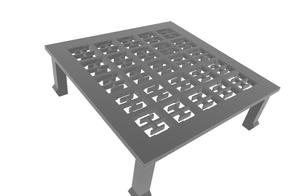3D-Mesh (cannot visualize here because of dimension constraint)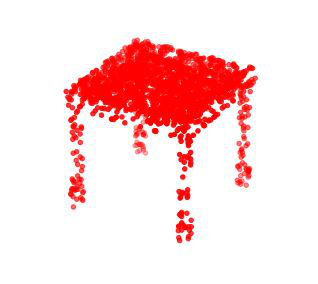Point cloud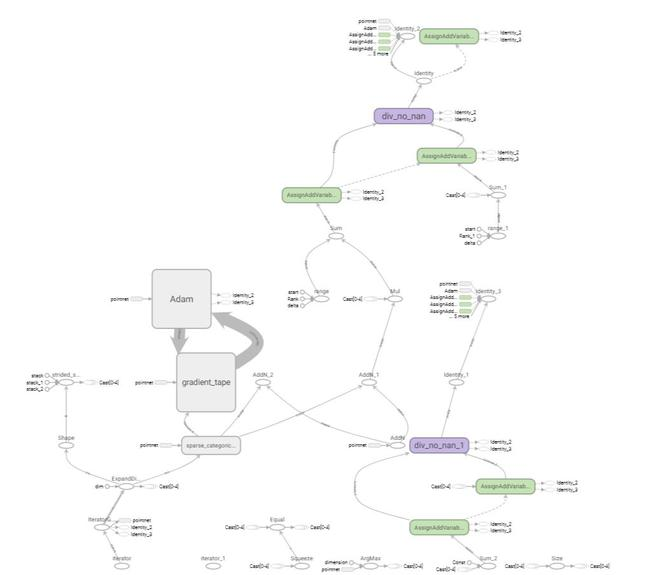TensorFlow Graph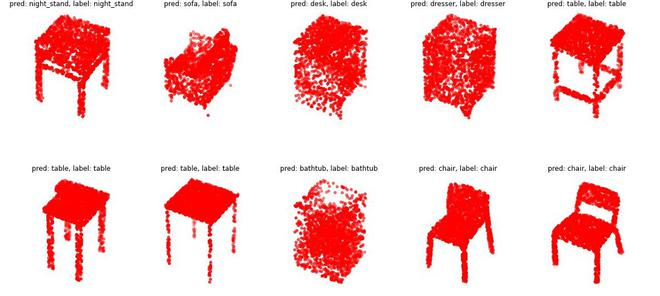Classification result

#### References:

Attention reader! Don’t stop learning now. Get hold of all the important Machine Learning Concepts with the Machine Learning Foundation Course at a student-friendly price and become industry ready.

My Personal Notes arrow_drop_up